11

Summary

Factorization

The number 11 is a prime number.

Properties and families

Property or family Parameter values First few numbers Proof of satisfaction/containment/membership
prime number fifth prime number 2, 3, 5, 7, 11, 13, 17, 19, 23, 29, 31, 37, 41, 43, 47, 53, 59, 61, 67, 71, 73, 79, 83, 89, 97, [SHOW MORE]View list on OEIS divide and check
safe prime (prime that is of the form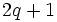for other prime) third safe prime 5, 7, 11, 23, 47, 59, 83, 107, 167, [SHOW MORE]View list on OEIS
Sophie Germain prime (primesuch that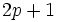is prime) fourth Sophie Germain prime 2, 3, 5, 11, 23, 29, 41, 53, 83, 89, [SHOW MORE]View list on OEIS
regular prime fourth regular prime 3, 5, 7, 11, 13, 17, 19, 23, 29, 31, 41, 43, 47, 53, 61, [SHOW MORE]View list on OEIS
lucky number of Euler fourth of six such numbers 2, 3, 5, 11, 17, 41 View list on OEISis of this kind iff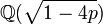has class number 1, i.e., its ring of integers is a unique factorization domain.

Structure of integers mod 11

Discrete logarithm

2 is a primitive root mod 11, so we can take it as the base of the discrete logarithm. We thus get an explicit bijection from the additive group of integers mod 10 to the multiplicative group of nonzero congruence classes mod 11, given by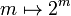. The inverse of that mapping is the discrete logarithm, i.e., the discrete logarithm ofismeans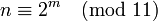:

Congruence class mod 11 (written as smallest positive integer) Congruence class mod 11 (written as smallest magnitude integer) Discrete logarithm to base 2, written as integer mod 10 Is it a primitive root mod 11 (if and only if the discrete log is relatively prime to 10)? Is it s quadratic residue or nonresidue mod 11 (residue if discrete log is even, nonresidue if odd)
1 1 0 No quadratic residue
2 2 1 Yes quadratic nonresidue
3 3 8 No quadratic residue
4 4 2 No quadratic residue
5 5 4 No quadratic residue
6 -5 9 Yes quadratic nonresidue
7 -4 7 Yes quadratic nonresidue
8 -3 3 Yes quadratic nonresidue
9 -2 6 No quadratic residue
10 -1 5 No quadratic nonresidue

Instead of 2, we could have taken 6, 7, or 8 as the base for discrete logarithm. The discrete logarithm thus obtained would differ from the original discrete logarithm via a multiplication by some suitable integer mod 10 (see base change for discrete logarithm).

Primitive roots

The number of primitive roots equals the number of generators of the additive group of integers mod 10, which is the Euler totient function of 10, which is 4. Given any primitive root, the primitive roots are those whose discrete log to baseis relatively prime to 10, In other words, they are the numbers. The explicit list of primitive roots is 2,6,7,8.

We note the following:

Of the ten congruence classes of invertible elements mod 11, five give quadratic residues and five give quadratic nonresidues. In terms of discrete logarithms, the quadratic residues correspond to even values and the quadratic nonresidues correspond to odd values of the logarithm. Explicitly, ifis a primitive root, the quadratic residues areand the nonresidues are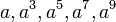.

Alternatively, the quadratic residues can be computed by taking the squares of the first five natural numbers and reducing them mod 11.

Explicitly, the quadratic residues are 1,3,4,5,9 and the quadratic nonresidues are 2,6,7,8,10.

Note that in this case, all the quadratic nonresidues other than 10 (which is -1 mod 11) are primitive roots. This follows from the fact that quadratic nonresidue that is not minus one is primitive root for safe prime.

Prime-generating polynomials

Below are some polynomials that give prime numbers for small input values, which give the value 11 for suitable input choice.

Polynomial Degree Some values for which it generates primes Input valueat which it generates 11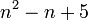2 all numbers 1,2,3,4, because 5 is one of the lucky numbers of Euler. 32 all numbers 1-10, because 11 is one of the lucky numbers of Euler. 1

Multiples

Interesting multiples

Number Prime factorization What's interesting about it
341 11 times 31 smallest Poulet number (also called Sarrus number), i.e., smallest Fermat pseudoprime to base 2
561 3 times 11 times 17 smallest Carmichael number, i.e., smallest absolute pseudoprime